Next: Coupled Oscillations Up: Damped and Driven Harmonic Previous: Transient Oscillator Response

# Exercises

1. Show that the period between successive zeros of a damped harmonic oscillator is constant, and is half the period between successive maxima.

2. Show that the ratio of two successive maxima in the displacement of a damped harmonic oscillator is constant. [From Fowles and Cassiday 2005.]

3. If the amplitude of a damped harmonic oscillator decreases to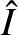of its initial value after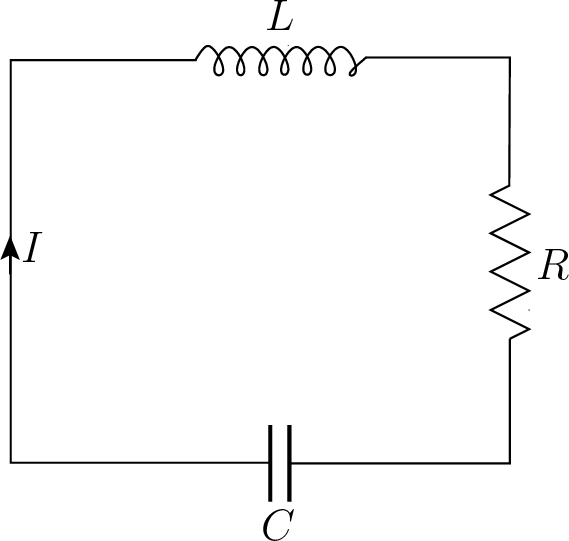periods show that the ratio of the period of oscillation to the period of the oscillation with no damping is approximately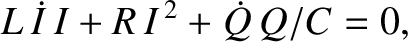[From Fowles and Cassiday 2005.]

4. Many oscillatory systems are subject to damping effects that are not exactly analogous to the frictional damping considered in Section 7. Nevertheless, such systems typically exhibit an exponential decrease in their average stored energy of the form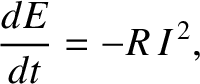. It is possible to define an effective quality factor for such oscillators as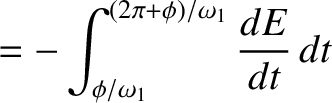, where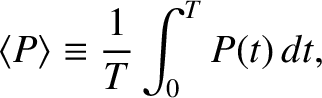is the natural angular oscillation frequency. For example, when the note middle C'' on a piano is struck its oscillation energy decreases to one half of its initial value in about 1 second. The frequency of middle C is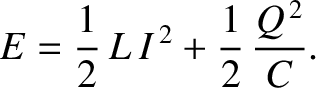Hz. What is the effective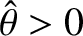of the system? [Modified from French 1971.]

5. According to classical electromagnetic theory, an accelerated electron radiates energy at the rate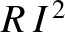, where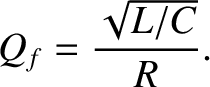,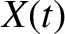is the charge on an electron,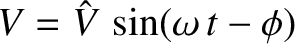the instantaneous acceleration, and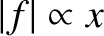the velocity of light in vacuum. If an electron were oscillating in a straight-line with displacement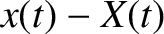how much energy would it radiate away during a single cycle? What is the effectiveof this oscillator? How many periods of oscillation would elapse before the energy of the oscillation was reduced to half of its initial value? Substituting a typical optical frequency (e.g., for green light) for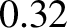, give numerical estimates for theand half-life of the radiating system.

6. Show that, on average, the energy of a damped harmonic oscillator of quality factor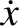decays by a factor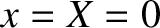duringoscillation cycles. By what factor does the amplitude decay in the same time interval?

7. Demonstrate that in the limit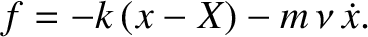the solution to the damped harmonic oscillator equation becomes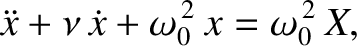where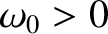and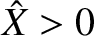.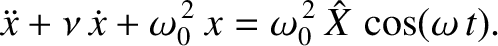8. What are the resonant angular frequency and quality factor of the circuit shown in the preceding figure? What is the average power absorbed at resonance?

9. The power input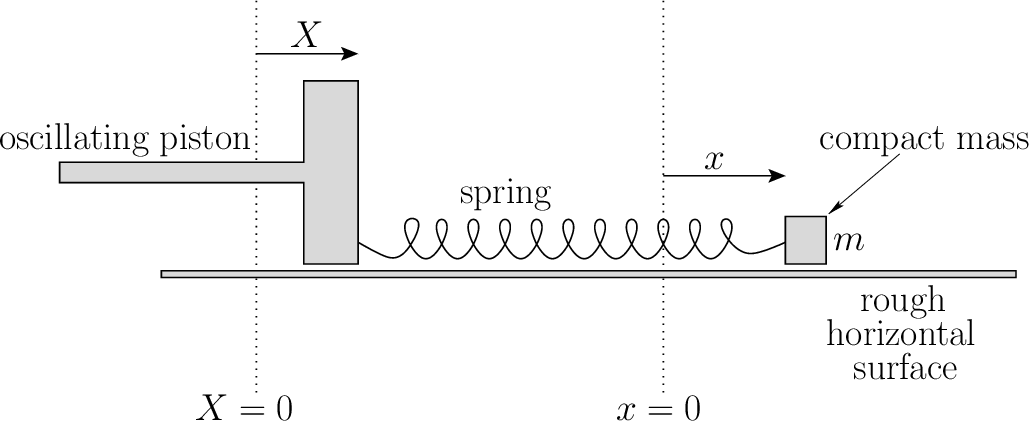required to maintain a constant amplitude oscillation in a driven damped harmonic oscillator can be calculated by recognizing that this power is minus the average rate that work is done by the damping force,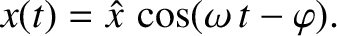.
1. Using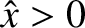, show that the average rate that the damping force does work is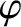.

2. Substitute the value of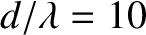at an arbitrary driving frequency and, hence, obtain an expression for.

3. Demonstrate that this expression yields (120) in the limit that the driving frequency is close to the resonant frequency.

10. The equation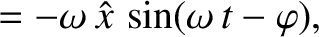governs the motion of an undamped harmonic oscillator driven by a sinusoidal force of angular frequency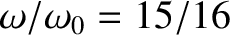. Show that the time asymptotic'' solution (i.e., the solution that would be time asymptotic were a small amount of damping added to the system) is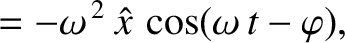where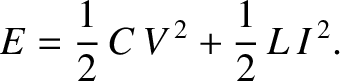. Sketch the behavior of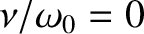versus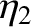for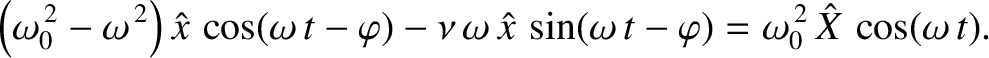and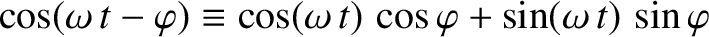. Demonstrate that if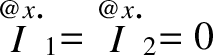atthen the general solution is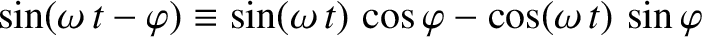Show, finally, that ifis close to the resonant frequencythen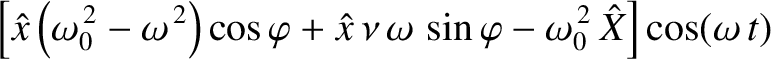Sketch the behavior ofversus. [Modified from Pain 1999.]Next: Coupled Oscillations Up: Damped and Driven Harmonic Previous: Transient Oscillator Response
Richard Fitzpatrick 2013-04-08